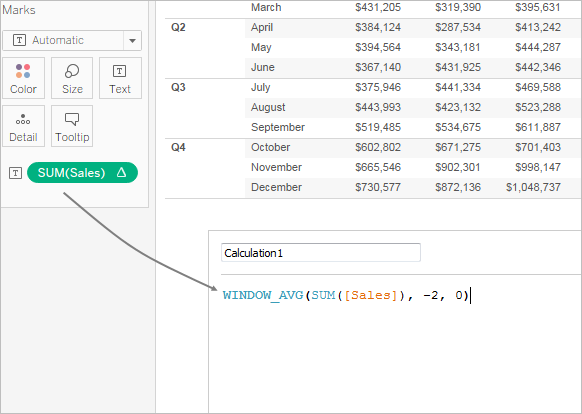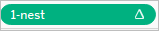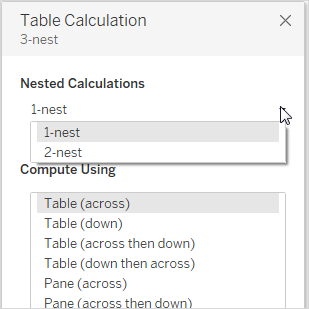You can always customize a table calculation by editing it in the Table Calculations dialog box, but there are other, more specialized ways to customize a table calculation.

## Customizing a Table Calculation Using Its Context Menu

Click any field in the view to see a context menu listing ways to customize the field. For a field in the view that has a table calculation, you can change the Compute Using option—that is, the option that determines the direction and scope of the calculation relative to the visual structure of the view. Do this by clicking the field and then choosing an option from the Compute Using list.

For Difference From, Percent Difference From, and Percent From table calculations, you can also specify a different field from which the difference should be computed. Do this by clicking the field and then choosing an option from the Relative to list. The options are Previous, Next, First, and Last.

## Customizing a Table Calculation Using the Calculation Editor

You can customize a table calculation by dragging it into the calculation editor:When you edit a table calculation in the calculation editor, you can click Default Table Calculation in the lower-right corner of the editor to open the calculation in the Table Calculation dialog box. This will allow you to create a new named calculated field that uses the same table calculation as the one you are basing the calculation on.

## Nested Table Calculations

A nested table calculation can be one of two types of calculated fields:

• A calculated field that includes more than one calculated field with a table calculation (as in the example below), or

• A calculated field that itself has a table calculation and includes at least one calculated field with a table calculation.

With nested table calculations, you can set Compute Using configurations for individual calculations independently.

Here is a scenario you can try, using the Sample - Superstore data source that is included with Tableau Desktop, which results in a nested table calculation.

1. Drag Sub-Category to Columns and Region to Rows.

2. Create a calculated field, 1-nest, with the definition `TOTAL(SUM([Sales]))`.

TOTAL is a table calculation function, so this calculated field automatically has a table calculation—when you use it in the view, the field will have the tell-tale table triangle, indicating a table calculation:For information on table calculation functions, see Table Calculation Functions.

3. Create a second calculated field, 2-nest, with the definition `TOTAL(SUM([Profit]))`.

4. Create a third calculated field, 3-nest, with the definition `[1-nest] + [2-nest]`.

5. Drag 3-nest and drop it to the right of `Sub-Category` on Columns.

6. Click 3-nest on Columns and choose Edit Table Calculation.

In the Table Calculations dialog box, you can now separately configure the underlying table calculations: